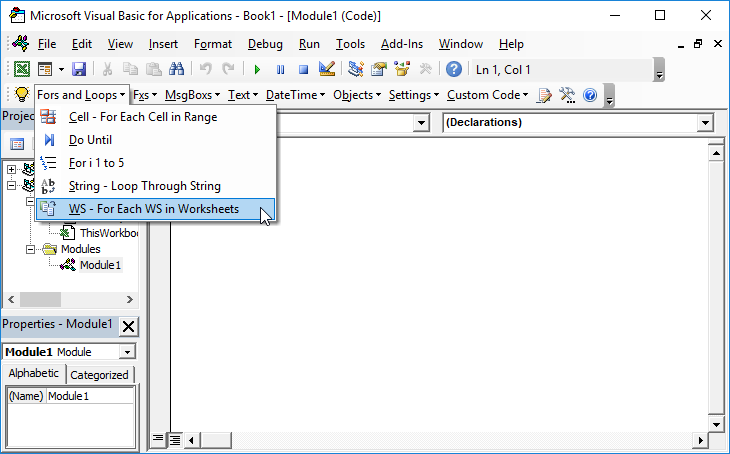# VBA – ActiveWindow.WindowState

## ActiveWindow.WindowState

There are three different WindowSates that a worksheet can have; Minimized, Maximized, and Normal. You can set the window state with one of these lines of code:

``````

ActiveWindow.WindowState = xlMinimized

ActiveWindow.WindowState = xlMaximized

ActiveWindow.WindowState = xlNormal

``````

And of course, once you can program a visual element you can throw in a loop and create an “animated effect”. The following would be an interesting effect possibly when unhiding a worksheet.

This macro will gradually resize a worksheet from small to Maximized, making the worksheet appear to be growing:

``````

Sub SheetGrow()

Dim x As Integer

With ActiveWindow

.WindowState = xlNormal

.Top = 1

.Left = 1

.Height = 50

.Width = 50

For x = 50 To Application.UsableHeight

.Height = x

Next x

For x = 50 To Application.UsableWidth

.Width = x

Next x

.WindowState = xlMaximized

End With

End Sub``````